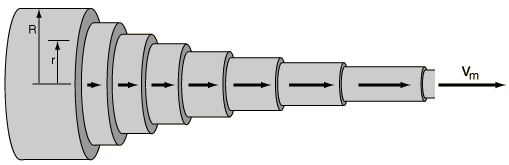## Monday, March 03, 2014

### Laminar Flow

If symmetry is to have ever existed,  and,  you return to the original state, problems enter the picture because you are introducing "some thing" to the system? For example, you can only back up so far. The question is what does this fifth dimensional perspective allow you? You know Gravity and light have been joined?

Yes, when you change visual perspective, what does a line look like, as in viewing a cylindrical system, with such a viscosity?

You cannot show where droplets were injected, and to go beyond that point of submersion, an example of what begin in rotation would on reversibility, happen same. So, something is missing?

My question is: could you ever learn the answer to an otherwise-intractable computational problem by jumping into a black hole?

Entanglement,  is not an option in such a system ? As is FTL, medium dependent? Changing viscosity rates show speed of light variance?

I want to discuss today reflect a different perspective: one that regards computation as no more “arbitrary” than other central concepts of mathematics, and indeed, as something that shows up even in contexts that seem incredibly remote from it, from the AdS/CFT correspondence to turbulent fluid flow. See:Recent papers by Susskind and Tao illustrate the long reach of computation
***Fluid Velocity Profile
Visualization above,  has specific destination in relation to specificity of drop,  as to show distance from center?

Kaluza-Klein theory is a model which unifies classical gravity and electromagnetism. It was discovered by the mathematician Theodor Kaluza that if general relativity is extended to a five-dimensional spacetime, the equations can be separated out into ordinary four-dimensional gravitation plus an extra set, which is equivalent to Maxwell's equations for the electromagnetic field, plus an extra scalar field known as the "dilaton". Oskar Klein proposed that the fourth spatial dimension is curled up with a very small radius, i.e. that a particle moving a short distance along that axis would return to where it began. The distance a particle can travel before reaching its initial position is said to be the size of the dimension. This, in fact, also gives rise to quantization of charge, as waves directed along a finite axis can only occupy discrete frequencies.

Kaluza-Klein theory can be extended to cover the other fundamental forces - namely, the weak and strong nuclear forces - but a straightforward approach, if done using an odd dimensional manifold runs into difficulties involving chirality. The problem is that all neutrinos appear to be left-handed, meaning that they are spinning in the direction of the fingers of the left hand when they are moving in the direction of the thumb. All anti-neutrinos appear to be right-handed. Somehow particle reactions are asymmetric when it comes to spin and it is not straightforward to build this into a Kaluza-Klein theory since the extra dimensions of physical space are symmetric with respect to left-hand spinning and r-hand spinning particles.
Also to further speculate.....

Oskar Klein proposed that the fourth spatial dimension is curled up in a circle of very small radius, i.e. that a particle moving a short distance along that axis would return to where it began. The distance a particle can travel before reaching its initial position is said to be the size of the dimension. This, in fact, also gives rise to quantization of charge, as waves directed along a finite axis can only occupy discrete frequencies. (This occurs because electromagnetism is a U(1) symmetry theory and U(1) is simply the group of rotations around a circle).

Placing comment here until approved  or not approved.

Instituting a experimental argument is necessary, when t comes to symmetry in the realtor of viscosity and entanglement? Light in Ftl is medium dependent?

This sets up analogue example of the question of firewalls as to imply Black holes and information?

Layman wondering.

***